# Are You Confusing kW With kWh? You’re Not Alone

The differencebetween kW (kilowatts) and kWh (kilowatt-hours) is one of the most misunderstood, and hence, widely discussed topics in the solar industry. However, energy literacy is not just a matter of semantics, but also economics.

The layperson can be forgiven for mixing up these two important metrics, but perhaps we shouldn’t be so lenient with solar salespeople, energy professionals, and journalists when talking all things electrical. Misusing these terms shows either a lack of understanding or care. Even a casual typo could end up being an expensive one.

Rather than deep diving into the technicalities of physics, this article will cover:

• A simple explanation of the terms kW (kilowatt) and kWh (kilowatt-hour).
• Examples of power and energy relating to appliances, household consumption, solar rooftops, home batteries, and electric vehicles.
• Why it helps to know the difference, and how it can save you money.

## kW And kWh – A Simple Explanation

SolarQuotes founder Finn Peacock has already written extensively on this subject1. These articles are still relevant and well worth reading. Here’s another take to help get the message through.

### kW (kilowatt)

kW (kilowatt) is a measure of power. Power in electrical terms it is the rate at which electrical energy is transferred in an electric circuit.

If an electrical appliance is powerful, its power rating (measured in kW) will be high, and it will consume electricity quickly.

### kWh (kilowatt-hour)

A kWh (kilowatt-hour) is a measure of energy. Energy in electrical terms is the ability or capacity to store and transfer that electricity over a period of time.

If you leave the above appliance on for a long time it will use a lot of energywhich can be measured in kWh.

### h (hour)

State the obvious but still relevant – an h (hour) is a measure of time. You already know what time it is.

In this case, we’re talking about the time the above appliance is running. We need to know this because it ties all the power and energy stuff together.

### Electrical Energy Equation

Here is a simple mathematical equation that shows the relationship between power (kW), energy (kWh)and time (h). Yes, it sounds boring, but stick with me, and you’ll be forever grateful.

Power (kW) x Time (h) = Energy (kWh)

That’s it. So simple it’s even in the name! kW x h = kWh. That’s all you need to know. I could finish here but I’ll keep rambling on and trying to empower you. I hope you have enough time to listen and don’t run out of energy (reader rolls eyes).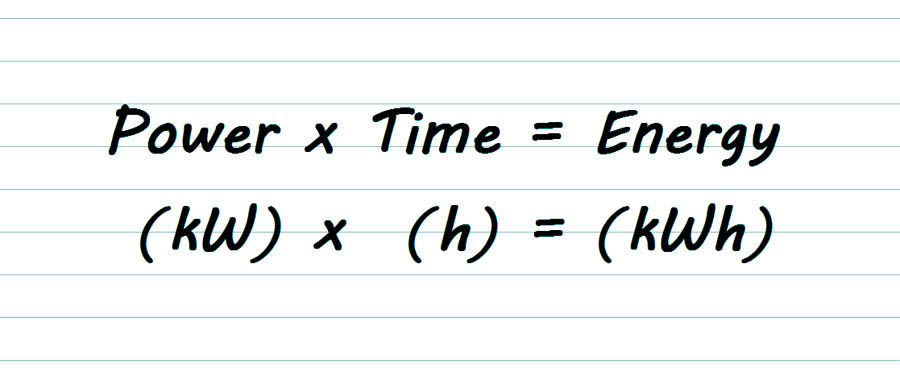## Examples of power and energy

### Electrical Appliance Example

In our appliance example above, let’s assume the power rating (on the nameplate) is 2kW, and the appliance runs for 4 hours. Using the electrical energy equation, it’s easy to see what the appliance uses 8kWh of energy.

Power (2kW) x Time (4h) = Energy (8kWh)

Shown on a graph, the Energy consumed is the area under the line.

It looks like this: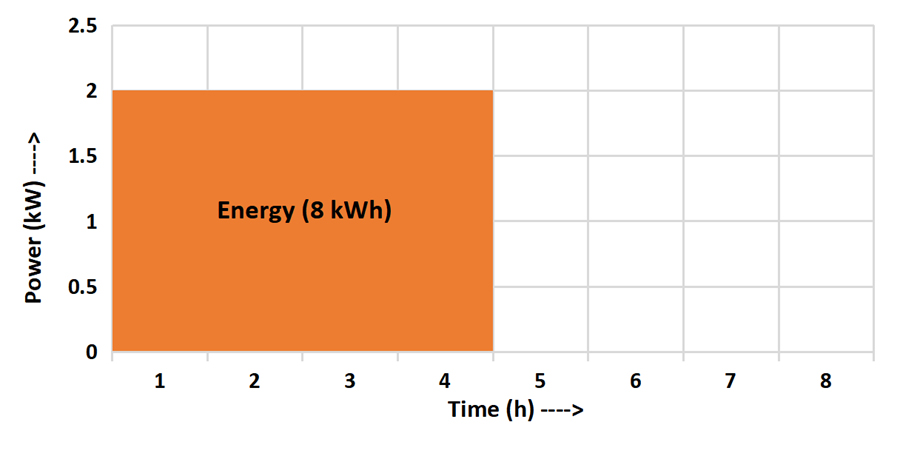Alike, an appliance rated at 1kW would use the same amount of energy (8kWh) if it ran for 8 hours.

Power (1kW) x Time (8h) = Energy (8kWh)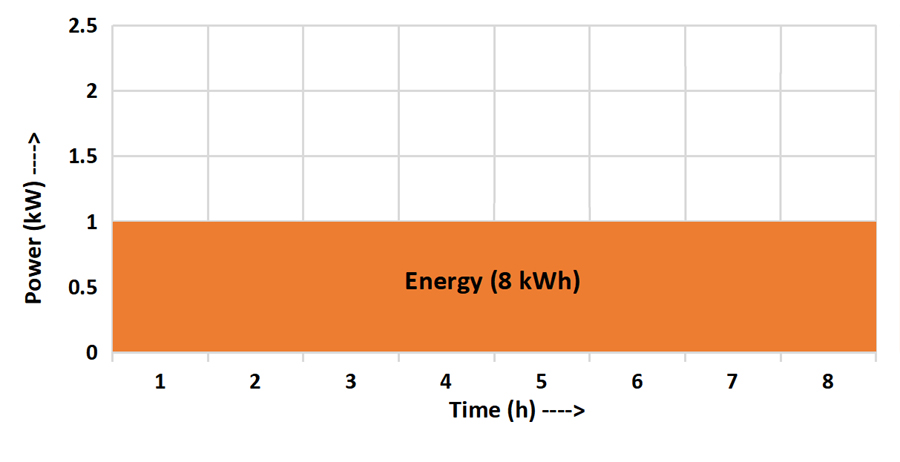### Electrical Appliance Example Take 2

Let’s muddy the waters a little. Although a nameplate may specify 2kWthat usually refers to peak power or the maximum rate it will consume energy. In reality, most electrical appliances use power at varying rates.

A washing machine may use different rates of power on different cycles, such as heating the water, washing, and spinning cycles. A full load will use more power than a half-full load. In this case (and in all cases), the electrical energy equation uses average power over the given time.

If an appliance is rated at 2kW peak power runs at 2kW for the first hour, 1kW for the second hour, 2kW for the third hour, and 1kW for the fourth hour, the average power would be 1.5kW (2kW + 1kW + 2kW + 1kW = 6kW divided by 4 hours)

The electrical energy equation would then be Power (1.5kW) x Time (4h) = Energy (6kWh)

Shown on a graph, it would look something like this: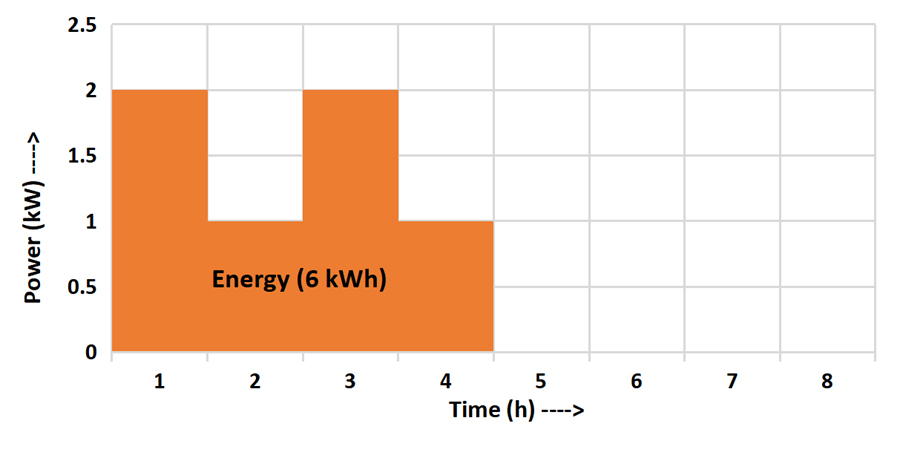Note that an appliance rarely runs at a particular power rating in neat little chunks of 1-hour blocks, as shown on the graph. This is only to get the point across.

### Household Energy Consumption Example

Your household uses many appliances that mostly run at varying rates of power at different times of the day. On top of that, many are constantly being switched on and off. Inputting the data into a mathematics equation would get complicated very quickly. It’s more easily shown on a graph or an app. This graph shows peak times of energy usage in the morning and evening.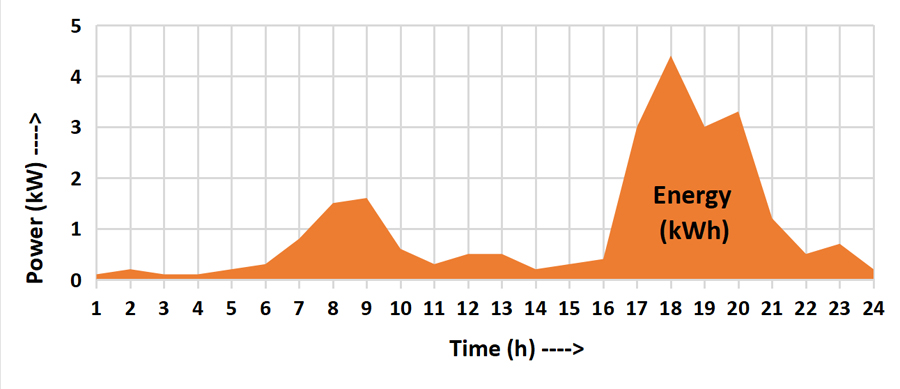Your energy provider usually charges you for the energy (kWh) you use over the billing period – not the power (kW). Notice I said *usually*. Hold that thought – more on that later.

### Rooftop Solar System Example

kW and kWh are used as a unit of measure for electricity generation and consumption. A rooftop solar power system, being a generator, is rated by the peak power of the solar array.

A solar array produces power at varying rates over a given time. This is because the output depends on factors such as location, weather, time of year, shading, efficiency, etc. It’s unlikely to hit the peak power (kW) for very long, if at all.

The following example uses a solar array rated at 5kW. You can see in the graph below that although the peak power is 5kWthe energy produced in 24 hours is NOT 5kW x 24hrs. That would be 120kWh! Instead, as in the previous examples, the calculation is based on average power over 24 hours:

0.2kW (average power) x 24hrs (time) = 20kWh (energy)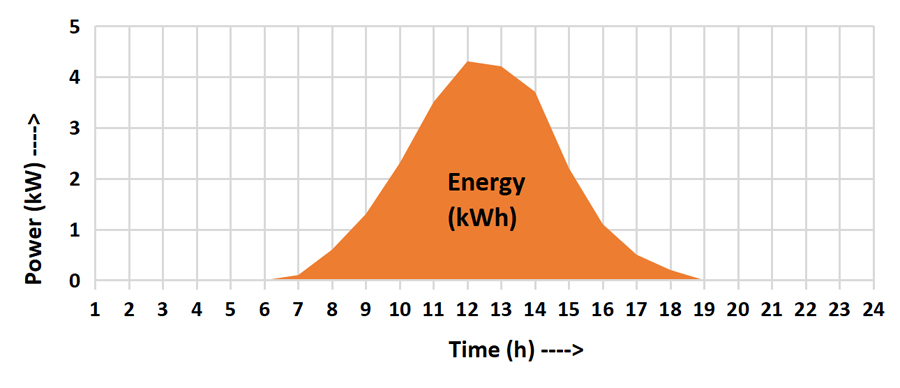Fortunately, thanks to satellites and some very clever people, there is good data available that will predict the likely solar energy available at any given location on Earth. They’ve worked out that if installed correctly, 1kW of solar power will produce approximately 4kWh of energy per day in most parts of Australia. Here’s another equation that gives the same result as above:

5kW (solar array peak power) x 4 (average energy per kW over 24 hours) = 20kWh (estimated energy)

### Home Battery Example

The size of a home battery is shown in kWh (kilowatt-hours). The is the usable energy or capacity of the battery. There is also a power rating if you dig into the datasheet. For example, a 10kWh battery may have a power rating of 5kW peak power. This means if it was discharging at the fastest possible rate (5kW) the 10kWh battery would be empty in 2 hours.

Of course, that rarely happens because batteries charge and discharge at varying rates. The following graph shows energy (kWh) flows both ways as a battery discharges, recharges, and discharges again over 24 hours. The battery SOC (state of charge) can be determined by adding up the energy in and out, and a whole bunch of other stuff that we’ll save for another day.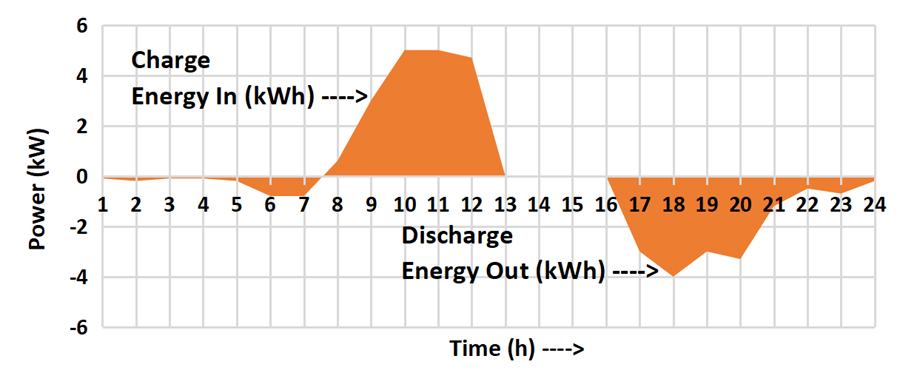### Electric Vehicle Example

EVs also have a power (kW) and an energy (kWh) specification. Obvious to you by now, the power rating relates to the motor and the energy capacity of the battery. EV buyers pay particular attention to the size of the fuel tank (battery), which is given in kWh. Most petrol car owners couldn’t even tell you the size of their fuel tank.

## Common Misuses Of The Terms kW (kilowatt) And kWh (kilowatt-hour)

Believe it or not, I’ve found the following statements and/or questions on blog comments and social media forums. Let me know if I can add any more to the list.

“My solar panels produced 18kW of electricity yesterday.”

Um, no they didn’t. They produced 18kWh of electricity yesterday.

Hello all, hoping to get some advice on this quote. It’s a small unit, using 5kW per day max.

The quote they got was for a 5kW rooftop solar system! This is a classic mistake that could cost this person big dollars for no good reason if they meant 5kWh per day. This is almost certainly what they meant because 5kW per day makes no sense, and 5kWh per day consumption, although low, is quite possible for 1 or 2 people in a small unit.

“The nameplate on my dryer has 2.4kW stamped on it. Does this mean I need to be generating 2.4kWh to run the machine?

The short answer is no. The person may or may not have interchanged the terms kW and kWh, so assumptions have to be made. If they are asking what the rate of power (kW) the machine uses, the answer is that it will pull 2.4kW maximum through its cycle but most likely average much less. If the person was asking about the energy (kWh) consumption the answer is still “no” because it depends on how long the dryer runs. Remember this simple equation, kWh = kW x h.

“If a solar panel produces 4kWh of power per day, does it mean that it produces 4kW of power every hour? If it is so it will generate 96kWh of energy for the whole day? Or is it producing 4kWh of energy in the whole day which means 0.166 kWh of energy every hour?”

She answered this for herself. The second guess was almost correct. It produces 4kWh of energy in the whole day, which means AN AVERAGE of 0.166 kWh of energy every hour.

“My daily household electricity consumption is 12 kilowatt hours per day.”

No, it’s not. It is 12 kilowatt-hours (kWh) per day. kilowatt/hour means kilowatts per hour. Kilowatt-hours is the term used to measure energy, being a product of kilowatts and hours. Kilowatt-hours and kilowatt-hours mean vastly different things.

## Tomatoes, Tomartos, kilowatts, kilowatt-hours, Who Cares?

Not me. You make your bed, you lay in it. Here are some reasons YOU should care though:

1. As pointed out above, if you get kW and kWh mixed up, you might buy a rooftop solar system 4 or 5 times larger than needed. That’s a multi-thousand-dollar mistake.
2. Most people buy solar and/or batteries for financial reasons, so minimizing the payback time is a priority. Maximizing self-consumption is the key, and the best way to do that is to understand how you use energy so you can use the right appliances at the right time.
3. Earlier in this article, I mentioned how your energy provider *usually* charges you for the energy (kWh) you use over the billing period – not the power (kW). Well, that’s not always the case. Many businesses and some residential customers also get charged for peak power usage. This is known as demand pricing. Avoid being moved to one of these plans if possible. Understanding your power and energy will help immensely.
4. The other reason it’s good to differentiate between kW and kWh is for your vanity. It can be quite embarrassing attempting to talk to people about your new solar and battery system, but only mumble meaningless technobabble.

On the other hand, if you want to drive electrical geeks up the wall, just ignore all the above. Good luck!

One more thing – a small one k for kilo, a capital W for Watts, and a small one h for hour. Thanks.

### footnotes

1. If you can’t get enough of this stuff, please read Finn’s excellent articles on kW, kWh, power and energy here and here, and most probably many other places.